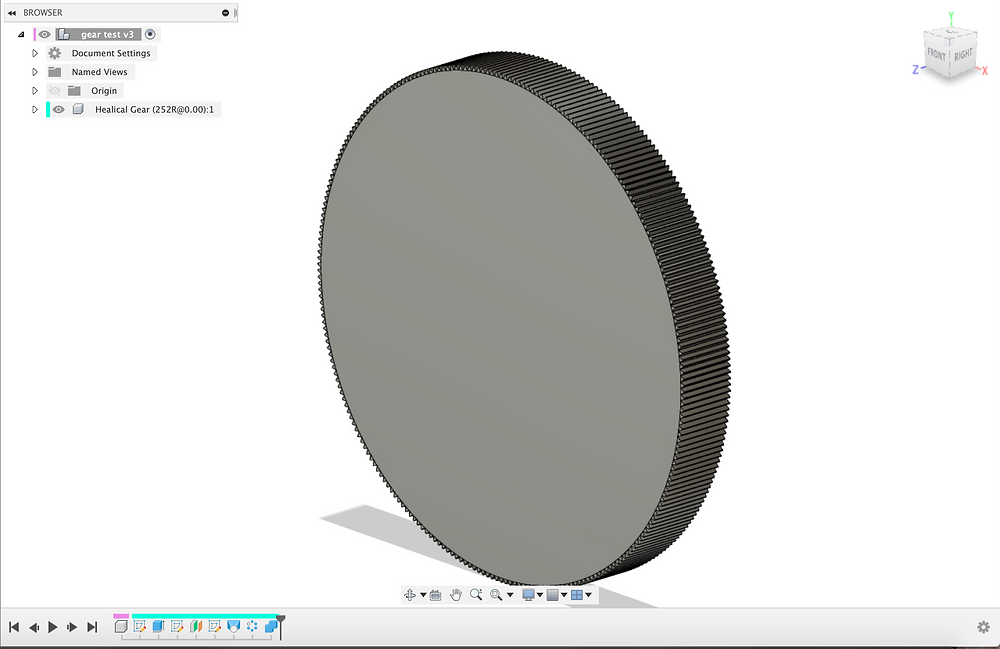Search
• aditya mehrotra.

# chip3 actuator updates: so we're going to try modeling the large gears, yes. [updates]

Yesterday we did a rough calculation and found out...

"

Let's start here with the shaft itself, we're going to download the cad and try to see if we can make a gear that will match this one. So we need to make a gear with MODULE = 0.5, PRESSURE ANGLE = 30, and then we're trying a gear ratio of 19:1 right so for a planetary that's...

(RING+STAR)/START = 19

so that's (RING+14)/14=19

RING = 252 teeth on this outer ring gear

"

We want to try to generate this gear in Fusion 360, and I think the new technique is to increase the data little by little till we get to a point fusion can handle the small level of computation instead of the regeneration of a huge gear file. I'm just going to be patient with this one until Fusion 360 asks me to leave and find a new CAD software. Remember, I'm just doing this as a test, because my brother is using my laptop for APs right now, I'll get that thing back after summer starts and then we get to do all of this in Solidworks!

So in the meantime, I want to see how big to make the planet gears, if the pitch diameter of the inner gear is 7mm, and we're going to find the pick diameter of the outer gear, then the pitch diameter of the planets must be:PDo/2-PDi/2 = PDp

Now once we know the PDp, we know the pitch circle is side:

p = pi*PDp

and then we can say the number of teeth is:

z = PDp/m

and

OH THE GEAR FINALL GENERATED YES. Let's do our math. The radius of this gear seems to be around 2.5in, so a diameter of ~5in which means yea our math was roughly right.So let's calculate the pitch diameter of this gear.

z=PDo/m; 252 = PDo/0.5; PDo = 126mm

Which means:

126/2-7/2=59.5mm for the PDp for the planetary gears

z = 59.5/0.5 --> 119 teethEach of the planets should have 119 teeth. Let's generate that gear now too so we don't miss out that. We're making all the gears 15mm thick. So we have the star gear in the form of the shaft of the Falcon 500, we have the planetary gears, and we have the ring-gear's form. That radius is 1.147in so it's looking pretty accurate so far.So one thing we can immediately see is that three gears is not going to work, this test assembly shows us that three gears would intersect with each other. So the planetary will end up looking like this:That's the Falcon 500 shaft in the middle, the 119 planetary gears around it, and the 252 tooth ring gear. We're going to have to make this assembly a little more "useful," and "properly meshed" before we get to anything else. We're going to have to actually make a ring gear and etc, but the point of this exercise wasn't to make a gearbox, it was to see if the ratios we were thinking were possible, if the shaft of the motor could be used as a gear, and you know what, it can be! We're going to determine further with testing if this is reasonable, we need to see what kind of torque that shaft is rated for and etc. We need to do a lot of verification but that will come with FEA and with some hand calculations. But at least this isn't completely unfeasible.

6 views0 comments

### Recent Posts

See All

#### chip updates: initial leg designs for cable drive test [updates]

© copyright 2019 | aditya mehrotra